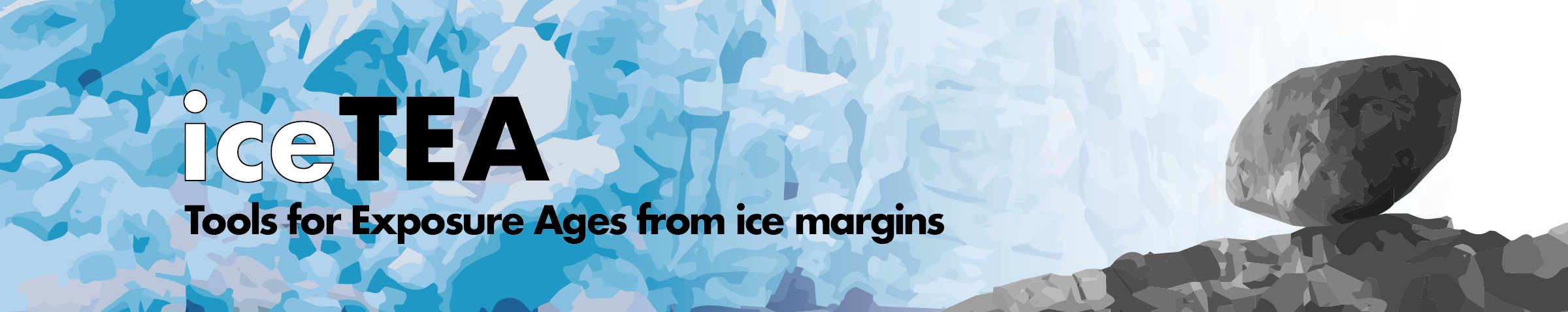## Correct for Surface Cover

The iceTEA tool determines shielding factors for material covering sample surfaces, and optionally calculates exposure ages correcting for this surface cover. The age calculation uses a modified version of the CRONUScalc calculation framework (Marrero et al., 2016) and global production rate calibration datasets (Borchers et al., 2016). The corrected ages can then be plotted as kernel density estimates.

Details are described here: Jones et al., 2019, Quaternary Geochronology (preprint version here).

Upload exposure age data in the ‘standard’ input form (as .xlsx, .csv or .txt). Then select a preset cover material or manually provide a density for the surface cover, as well as the cover depth. Optionally calculate the corresponding exposure ages using a preferred time-dependent production scaling model: ‘Lm’ (Lal, 1991); ‘LSD’ (Lifton et al., 2014); ‘LSDn’, nuclide-specific scaling (Lifton et al., 2014).